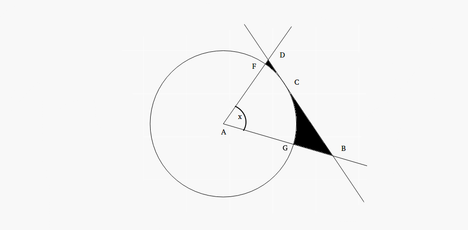# Practice on Toph

Participate in exhilarating programming contests, solve unique algorithm and data structure challenges and be a part of an awesome community.

# Circle Mystery

By ovis96 · Limits 1s, 512 MBHere, $A$ is the center of the circle and $BCD$ is a tangent which intersects with the circle at the point $C$. Moreover, the tangent intersects with the rays $\overrightarrow{\rm AF}$ and $\overrightarrow{\rm AG}$.

You are given the radius of the circle $r$, and the angle of the circular sector $x$. You have to minimize the area of the shaded region by choosing the point $C$ optimally. Note that the point $C$ must need to be inside the angle $\angle FAG$ and obviously on the circumference of the circle.

## Input

The first line of the input contains a single integer $t(1 \le t \le 100)$ denoting the number of test cases. The description of $t$ test cases follows.

The first and only line of each test case contains two space-separated integers $r(1 \le r \le 1000)$ and $x(1 \le x \le 90)$ — the radius of the circle and the angle of the circular sector in degrees respectively.

## Output

For each test case, output the minimum area of the shaded region that can be achieved by choosing the point $C$ optimally.

Your answer will be considered correct if its relative or absolute error doesn't exceed $10^{-6}$.

## Sample

InputOutput
3
3 10
45 45
96 69

0.0019998083
43.5668233656
784.6720511715


### Statistics

93% Solution Ratio

Roman_Emper0rEarliest, 9M ago

Roman_Emper0rFastest, 0.0s

RUHRUHLightest, 0 B

MursaleenShortest, 264B

### Submit